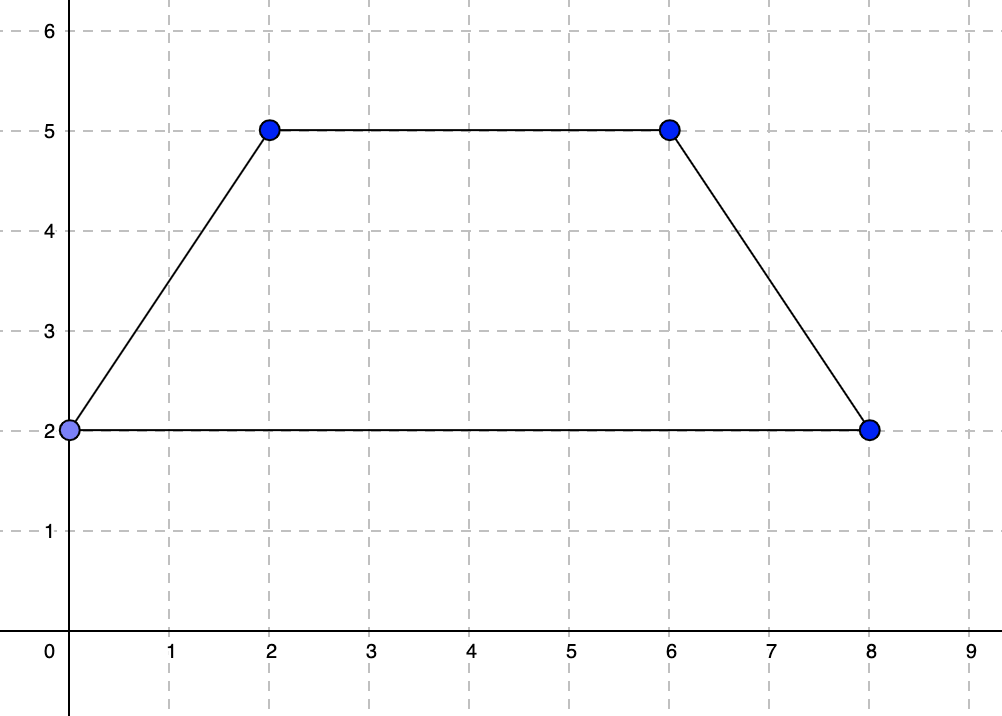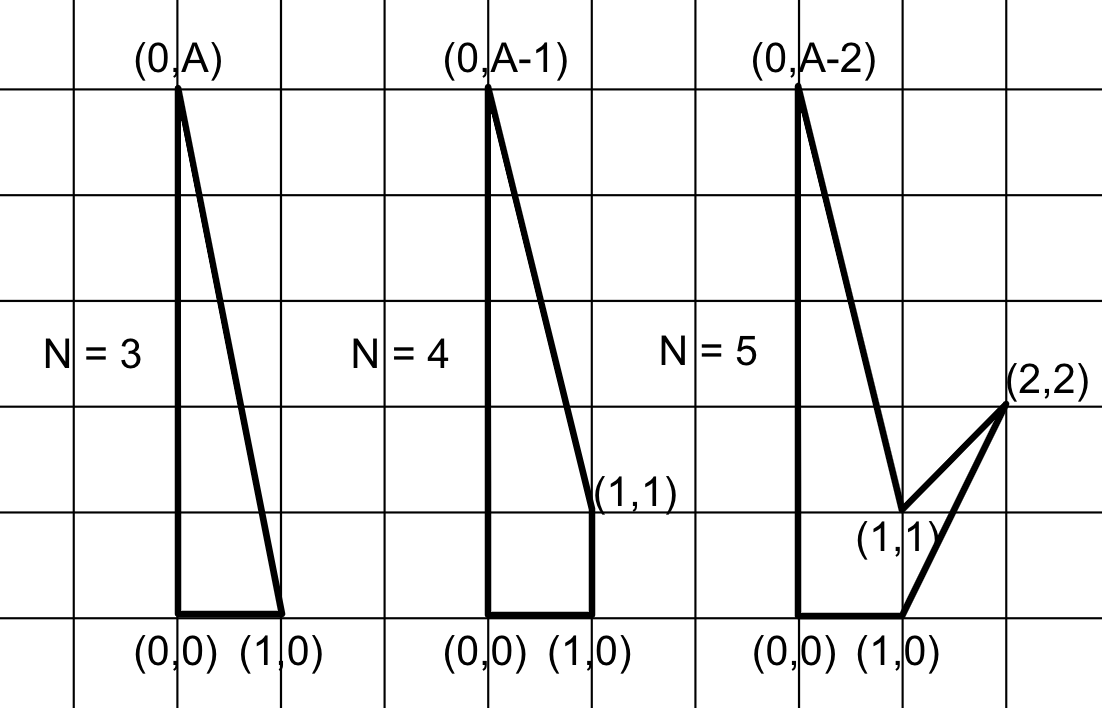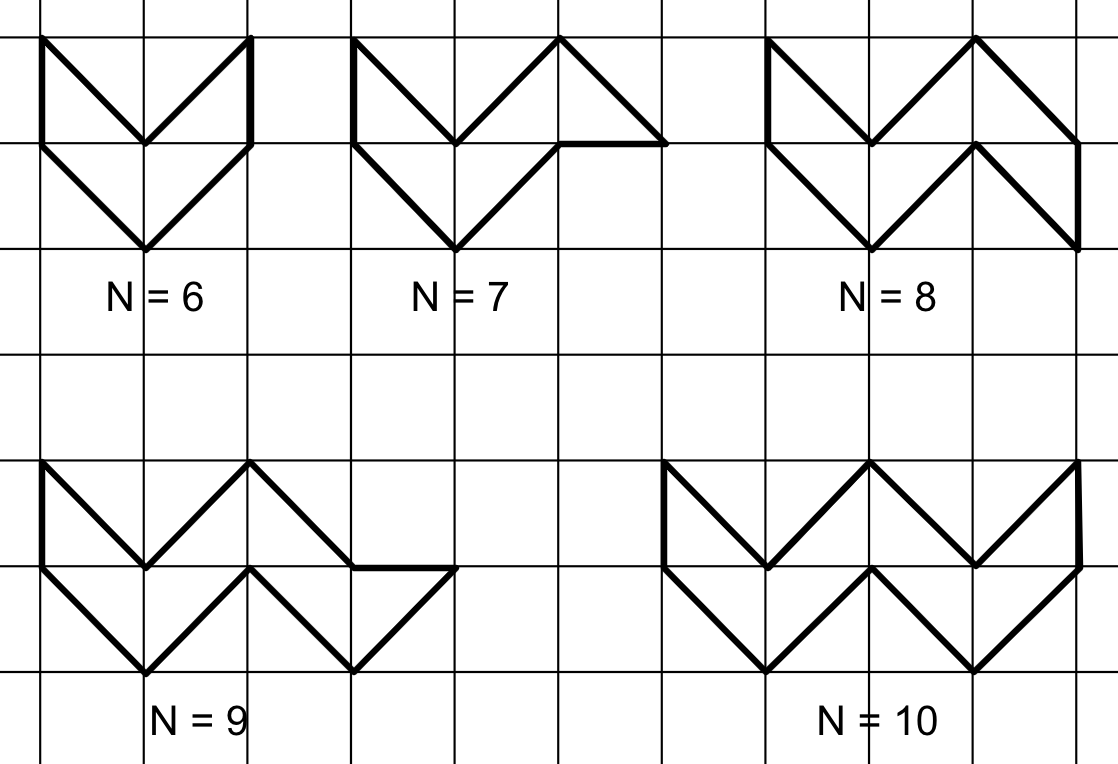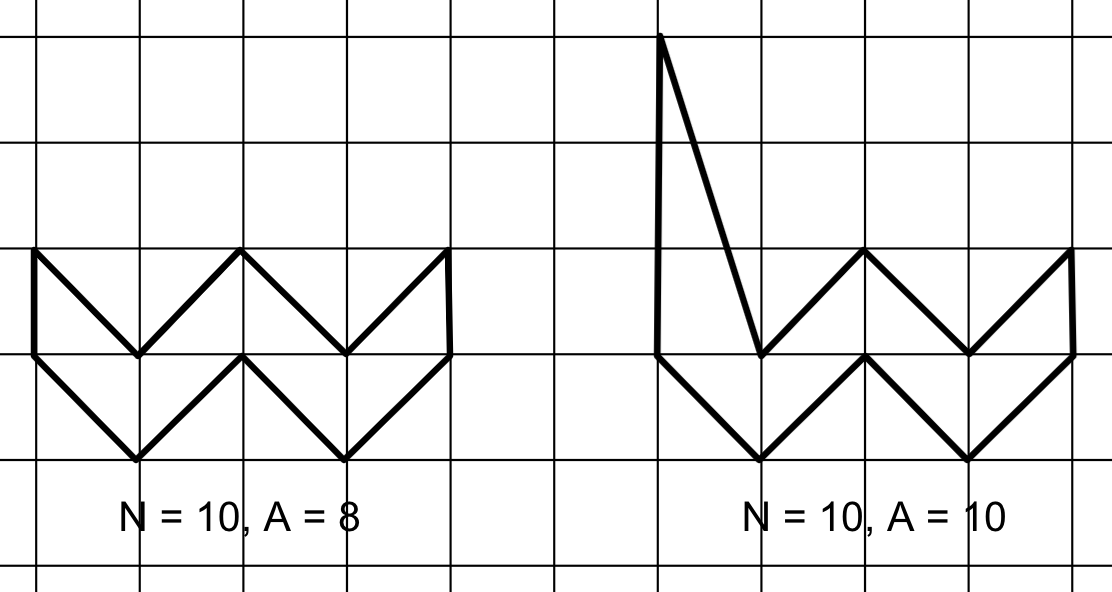# Google Kick Start Archive — Round G 2021 problems

## Overview

Thank you for participating in Kick Start 2021 Round G!

Cast

Dogs and Cats: Written by Sumedha Agarwal and prepared by Chu-ling Ko.

Staying Hydrated: Written by Deepika Naryani and prepared by Gagan Kumar.

Banana Bunches: Written by Claire Yang and prepared by Anson Ho.

Simple Polygon: Written by Pablo Heiber and prepared by Mahmoud Ezzat.

Solutions, other problem preparation, reviews and contest monitoring by Abhishek Singh, Akul Siddalingaswamy, Alan Lou, Anson Ho, Anurag Singh, Bartosz Kostka, Bohdan Pryshchenko, Chu-ling Ko, Chun-nien Chan, Claire Yang, Cristhian Bonilha, Dee Guo, Deeksha Kaurav, Deep Chowdhury, Deepak Gour, Deepika Naryani, Diksha Saxena, Gagan Kumar, Ishank Bhardwaj, Ishita Mundhra, Jared Gillespie, Kashish Bansal, Krists Boitmanis, Lizzie Sapiro Santor, Lucas Maciel, Mahmoud Ezzat, Maneeshita Sharma, Michał Łowicki, Mo Luo, Pablo Heiber, Phil Sun, Pranjal Jain, Rahul Goswami, Rathin Bhargava, Rishabh Agarwal, Ruoyu Zhang, Samiksha Gupta, Sangeeta Mishra, Sara Biavaschi, Sarah Young, Sasha Fedorova, Sharath Holla, Shweta Karwa, Sumedha Agarwal, Swapnil Gupta, Swapnil Mahajan, Teja Vardhan Reddy Dasannagari, Umang Goel, Vasyl Franchuk, Vijay Krishan Pandey.

Analysis authors:

• Dogs and Cats: Vijay Krishan Pandey
• Staying Hydrated: Krists Boitmanis
• Banana Bunches: Swapnil Gupta
• Simple Polygon: Krists Boitmanis

## A. Dogs and Cats

### Problem

You work for an animal shelter and you are responsible for feeding the animals. You already prepared $$\mathbf{D}$$$portions of dog food and $$\mathbf{C}$$$ portions of cat food.

There are a total of $$\mathbf{N}$$$animals waiting in a line, some of which are dogs and others are cats. It might be possible that all the animals in the line are dogs or all the animals are cats. A string $$\mathbf{S}$$$ of $$\mathbf{N}$$$characters C and D represents the order of cats and dogs in the line. The $$i$$$-th character is equal to C if the $$i$$$-th animal in the line is a cat. Similarly, the $$i$$$-th character is equal to D if the $$i$$$-th animal in the line is a dog. The animals are fed in the order they stay in the line. Each dog eats exactly $$1$$$ portion of dog food and similarly each cat eats exactly $$1$$$portion of cat food. Moreover, you have extra portions of cat food. Every time a dog eats food, you bring $$\mathbf{M}$$$ extra portions of cat food for cats.

Animals have to be fed in the order they wait in line and an animal can only eat if the animal before it has already eaten. That means that if you run out of dog (or cat) food portions and a dog (or a cat) is about to get fed, the line will not move, as all the animals will wait patiently.

You need to determine if in this scenario all the dogs in the line will be fed. Note that this means that some cats might remain in the line, but worry not, you will eventually feed them later!

### Input

The first line of the input gives the number of test cases, $$\mathbf{T}$$$. $$\mathbf{T}$$$ test cases follow.

The first line of each test case contains four integers $$\mathbf{N}$$$, $$\mathbf{D}$$$, $$\mathbf{C}$$$, and $$\mathbf{M}$$$: the number of animals, the initial number of dog food portions, the initial number of cat food portions, and the additional portions of cat food that we add after a dog eats a portion of dog food, respectively.

The next line contains a string $$\mathbf{S}$$$of length $$\mathbf{N}$$$ representing the arrangement of animals.

For each test case, output one line containing Case #$$x$$$: $$y$$$, where $$x$$$is the test case number (starting from $$1$$$) and $$y$$$is YES if all the dogs will be fed and NO otherwise. ### Limits Memory limit: 1 GB. $$1 \le \mathbf{T} \le 100$$$.
$$1 \le \mathbf{N} \le 10^4$$$. $$0 \le \mathbf{D}, \mathbf{C} \le 10^6$$$.

#### Test Set 2

Time limit: 40 seconds.
$$0 \le \mathbf{M} \le 10^6$$$. ### Sample Note: there are additional samples that are not run on submissions down below. Sample Input 3 6 10 4 0 CCDCDD 4 1 2 0 CCCC 4 2 1 0 DCCD  Sample Output Case #1: YES Case #2: YES Case #3: NO  In Sample Case #1, there are $$10$$$ portions of dog food and $$4$$$portions of cat food. 1. The first two animals are cats, so after they eat, $$2$$$ portions of cat food remain.
2. Then a dog eats one portion of dog food. Now, there are $$9$$$portions of dog food left. 3. Next, a cat eats a portion of cat food, reducing the number of portions of cat food to $$1$$$.
4. The last two animals are dogs and they each eat one portion of dog food.
So in this case, all the dogs are able to eat.

In Sample Case #2, there are no dogs. Hence, all (zero) dogs will be able to eat trivially.

In Sample Case #3, the cat before the second dog will not be able to eat because there will not be enough portions of cat food. Hence, the second dog will also not eat.

### Additional Sample - Test Set 2

The following additional sample fits the limits of Test Set 2. It will not be run against your submitted solutions.
Sample Input
2
12 4 2 2
CDCCCDCCDCDC
8 2 1 3
DCCCCCDC

Sample Output
Case #1: YES
Case #2: NO


In Sample Case #1, $$2$$$portions of cat food appear whenever a dog eats a portion of dog food. 1. After the first cat eats, there is $$1$$$ portion of cat food left.
2. Then a dog eats, leaving $$3$$$portions of dog food and $$3$$$ portions of cat food.
3. After the next $$3$$$cats eat, there are $$3$$$ portions of dog food and $$0$$$portions of cat food remaining. 4. Then a dog eats, leaving $$2$$$ portions of dog food and $$2$$$portions of cat food. 5. After the next $$2$$$ cats eat food, there are $$2$$$portions of dog food and $$0$$$ portions of cat food left.
6. Now a dog eats, leaving $$1$$$portion of dog food and $$2$$$ portions of cat food.
7. Next a cat eats, leaving $$1$$$portion of dog food and $$1$$$ portion of cat food.
8. The last dog eats the remaining portion of dog food.
So in this case, all the dogs are able to eat.

In Sample Case #2, the cat before the second dog will not be able to eat because there will not be enough portions of cat food.

## B. Staying Hydrated

### Problem

With online classes in full swing, it is important for Grace to take breaks and keep herself hydrated at all times. She has decided to place a water bottle in her room in the most convenient place. This means that the position of this water bottle should be close to all the places in the room where she generally hangs out like the study desk, bed and coffee table among other places.

The room is represented in the form of a coordinate plane. The number of steps Grace needs to go from Point A to Point B is equal to the Manhattan distance between the 2 points. This means, Grace can only walk parallel to the axes of the coordinate plane and with each step, she can move one unit in either of the four directions.

Can you help her find a position in the room to keep the bottle, such that the sum of steps from the bottle to all her favourite furniture pieces will be minimum?

Notes:

• All the furniture (like study desk, bed, or coffee table) can be represented as rectangles of non-zero area in the plane with edges parallel to the axes.
• It is possible for furniture pieces to overlap, as she likes to work on her bed-table too.
• Assume that Grace can simply pass through the furniture while walking and does not need to go around them.
• ### Input

The first line of the input gives the number of test cases, $$\mathbf{T}$$$. $$\mathbf{T}$$$ test cases follow.
The first line of each test case contains an integer $$\mathbf{K}$$$which represents the number of objects in Grace's room. $$\mathbf{K}$$$ lines follow, each of them describing one object. The $$i$$$-th line contains four integers, $$\mathbf{x_{i,1}}$$$, $$\mathbf{y_{i,1}}$$$, $$\mathbf{x_{i,2}}$$$, $$\mathbf{y_{i,2}}$$$, where ($$\mathbf{x_{i,1}}$$$, $$\mathbf{y_{i,1}}$$$) represents coordinates of the bottom left corner and ($$\mathbf{x_{i,2}}$$$, $$\mathbf{y_{i,2}}$$$) represents coordinates of the top right corner of the $$i$$$-th rectangular object.

### Output

For each test case, output one line containing Case #$$i$$$: $$x$$$ $$y$$$, where $$i$$$ is the test case number (starting from 1) and $$x$$$and $$y$$$ are coordinates of the water bottle such that the sum of steps from these coordinates to all the furniture pieces will be minimum.
Note, the bottle can lie on the floor or on top of any furniture but should be placed on integer coordinates only.
If multiple solutions exist, output the one with minimum x coordinate, if multiple solutions have the same x coordinate output the one with minimum y coordinate.

### Limits

Memory limit: 1 GB.
$$1 \le \mathbf{T} \le 100$$$. $$\mathbf{x_{i,1}} \lt \mathbf{x_{i,2}}$$$, for all $$i$$$. $$\mathbf{y_{i,1}} \lt \mathbf{y_{i,2}}$$$, for all $$i$$$. #### Test Set 1 Time limit: 40 seconds. $$1 \le \mathbf{K} \le 20$$$.
$$-100 \le \mathbf{x_{i,1}},\mathbf{x_{i,2}},\mathbf{y_{i,1}},\mathbf{y_{i,2}} \le 100$$$, for all $$i$$$.

#### Test Set 2

Time limit: 90 seconds.

For each test case, output one line containing Case #$$x$$$: $$y$$$, where $$x$$$is the test case number (starting from $$1$$$) and $$y$$$is the minimum number of trees Barbara must purchase to obtain $$\mathbf{K}$$$ banana bunches using at most $$2$$$contiguous sections of the farm, or -1 if it is impossible to do so. ### Limits Time limit: 20 seconds. Memory limit: 1 GB. $$1 \le \mathbf{T} \le 100$$$.
$$0 \le \mathbf{B_{i}} \le \mathbf{K}$$$, for each $$i$$$ from $$1$$$to $$\mathbf{N}$$$.

#### Test Set 1

$$1 \le \mathbf{K} \le 10^4$$$. $$1 \le \mathbf{N} \le 50$$$.

#### Test Set 2

$$1 \le \mathbf{K} \le 10^4$$$. $$1 \le \mathbf{N} \le 500$$$.

#### Test Set 3

$$1 \le \mathbf{K} \le 10^6$$$. For at most 25 cases: $$1 \le \mathbf{N} \le 5000$$$.

For the remaining cases:
$$1 \le \mathbf{N} \le 500$$$. ### Sample Sample Input 4 6 8 1 2 3 1 2 3 4 10 6 7 5 2 6 8 3 1 2 1 3 1 4 6 3 1 2 0  Sample Output Case #1: 3 Case #2: -1 Case #3: 4 Case #4: 3  In Sample Case #1, the first section can contain the trees at indices $$2$$$ and $$3$$$, and the second section can contain the tree at index $$6$$$.

In Sample Case #2, it is impossible to achieve a sum of $$10$$$with $$2$$$ contiguous sections.

In Sample Case #3, the first section can contain the trees at indices $$\{1, 2\}$$$, and the second section can contain the trees at indices $$\{5, 6\}$$$. We cannot take the $$2 + 3 + 3$$$combo (trees at indices $$\{1, 3, 5\}$$$) since that would be $$3$$$contiguous sections. In Sample Case #4, the only section contains the trees at indices $$\{1, 2, 3\}$$$.

## D. Simple Polygon

You are given two integers, the number of vertices $$\mathbf{N}$$$and area $$\mathbf{A}$$$. You need to construct a simple polygon of $$\mathbf{N}$$$vertices such that the area of the polygon is exactly $$\frac{\mathbf{A}}{2}$$$, and all the vertices have non-negative integer coordinates with value up to $$10^9$$$. A simple polygon is one that: • Defines a closed area. • Does not have self-intersections, even at a single point. • No two consecutive edges form a straight angle. • ### Input The first line of the input gives the number of test cases, $$\mathbf{T}$$$. $$\mathbf{T}$$$lines follow. The first line of each test case contains two integers, $$\mathbf{N}$$$ denoting the number of vertices and $$\mathbf{A}$$$, denoting double the required area of the polygon. ### Output For each test case, output one line containing Case #$$x$$$: $$y$$$, where $$x$$$ is the test case number (starting from 1) and $$y$$$is IMPOSSIBLE if it is not possible to construct a polygon with the given requirements and POSSIBLE otherwise. If you output POSSIBLE, output $$\mathbf{N}$$$ more lines with $$2$$$integers each. The $$i$$$-th line should contain two integers $$X_{i}$$$and $$Y_{i}$$$ which denote the coordinates of the $$i$$$-th vertex. For each $$i$$$, the coordinates should satisfy the $$0 \le X_{i}, Y_{i} \le 10^9$$$constraints. Vertices of the polygon should be listed in consecutive order ( $$vertex_{i}$$$ should be adjacent to $$vertex_{i-1}$$$and $$vertex_{i+1}$$$ in the polygon).

If there are multiple possible solutions, you can output any of them.

### Limits

Memory limit: 1 GB.
$$1 \le \mathbf{T} \le 100$$$. $$1 \le \mathbf{A} \le 10^9$$$.

#### Test Set 1

Time limit: 20 seconds.

### Sample

Sample Input
2
4 36
5 2

Sample Output
Case #1: POSSIBLE
2 5
6 5
8 2
0 2
Case #2: IMPOSSIBLEIn Sample Case #1, we can output the above quadrilateral with coordinates $$(2, 5)$$$, $$(6, 5)$$$, $$(0, 2)$$$and $$(8, 2)$$$. The area of this quadrilateral is equal to $$18$$$. In Sample Case #2, there is no way to construct a polygon with $$5$$$ vertices and area equal to $$1$$$. ## Analysis — A. Dogs and Cats We need to determine if all the dogs will be able to eat food given the condition that an animal can only eat if the animal before it has already eaten. We do not worry if some cats are hungry as long as all the dogs are able to eat. Let us assume that the last (or rightmost) dog is at position $$p$$$. We do not need to worry about the cats in positions $$[p + 1, N]$$$. Since an animal can only eat food if the animal before it has already eaten, we need to make sure that all cats and dogs in positions $$[1, p]$$$ are able to eat food.

### Test Set 1

We are given $$\mathbf{M}$$$$$= 0$$$. This means whenever a dog eats food, no cat foods magically appear. If $$\mathbf{S}$$$contains all $$0$$$ i.e., no dog is present, the answer is YES. Otherwise, we find the position of the last (or rightmost) dog, $$p$$$. Let the total number of dogs i.e., the number of $$1$$$ in $$\mathbf{S}$$$be $$X$$$ and the number of cats before the rightmost dog i.e., the number of $$0$$$in $$[1, p - 1]$$$ be $$Y$$$. The answer is YES if $$\mathbf{D} \ge X$$$ and $$\mathbf{C} \ge Y$$$and NO otherwise. Complexity : $$O(\mathbf{N})$$$ per test case

### Test Set 2

We are given $$\mathbf{M} \ge 0$$$. This means whenever a dog eats food, $$\mathbf{M}$$$ cat food portions are added. We iterate over positions $$[1,N]$$$and whenever we see a cat we feed it with the cat food i.e., subtract $$1$$$ from $$\mathbf{C}$$$and whenever a dog appears we feed it with the dog food, i.e., subtract $$1$$$ from $$\mathbf{D}$$$. We need to ensure that the dog can only eat if the animal before it has already eaten, and to do so, we first make sure that $$\mathbf{D} \ge 1$$$, as $$1$$$food item is needed to feed this dog and $$\mathbf{C} \ge 0$$$. If this is not true, our answer is NO. Otherwise we subtract $$1$$$from $$\mathbf{D}$$$ and add $$\mathbf{M}$$$to $$\mathbf{C}$$$. If the above condition is satisfied for all the dogs, our answer is YES. Note that this algorithm will also work for Test Set $$1$$$but not vice versa. ##### Sample Code(C++)  bool have_dogs_eaten(string S, int N, int D, long long C, int M) { for(int i = 0; i < N; i++) { if (S[i] == '1') { if(D <= 0 || C < 0) { return false; } D--; C += M; } else { C--; } } return true; }  Note that variable C is long long since maximum possible value does not fit in 32-bit data type. Complexity : $$O(\mathbf{N})$$$ per test case

Test Data
info We recommend that you practice debugging solutions without looking at the test data.

## Analysis — B. Staying Hydrated

### Test Set 2

In this test set, the furnished area of the room may be huge, therefore, even the improved version of the brute-force algorithm would be too slow. However, with a little bit of sorting, we can find the optimal location of the bottle without ever computing any distances.

Let us consider finding the optimal $$x$$$-coordinate of the bottle (the optimal $$y$$$-coordinate can be found in the same way). Imagine that we are seeking the optimal location by sweeping the coordinate plane from left to right. Suppose we are currently at the position $$x$$$and let $$a(x)$$$ be the number of rectangles that are strictly ahead of $$x$$$, i.e. $$x \lt x_1$$$, where $$x_1$$$is the left coordinate of the rectangle. Simlarly, let $$b(x)$$$ be the number of rectangles that are behind $$x$$$in the non-strict sense, namely, $$x_2 \le x$$$. Now, if $$a(x) \gt b(x)$$$, then $$x$$$ is not the optimal location as moving one step to the right would reduce the total horizontal distance to the rectangles by $$a(x)-b(x) \gt 0$$$. What happens to the value of $$a(x)-b(x)$$$ as we sweep the plane from left to right? For a sufficiently small $$x$$$, which is strictly to the left from all rectangles, $$a(x)=\mathbf{K}$$$ and $$b(x)=0$$$, and therefore, $$a(x)-b(x) = \mathbf{K} \gt 0$$$. Conversely, for a sufficiently large $$x$$$, it is the other way around and $$a(x)-b(x) = -\mathbf{K} \lt 0$$$. And since $$a(x)$$$is a decreasing function while $$b(x)$$$ is an increasing function, the difference $$a(x)-b(x)$$$is also a decreasing function. What does it mean for our plane sweeping approach? As long as $$a(x)-b(x) \gt 0$$$, we should keep moving to the right as, by doing so, we are reducing the total distance to the rectangles. But as soon as $$a(x)-b(x) \le 0$$$, we have found the optimal $$x$$$-coordinate, since further reduction of the total distance is not possible.

In summary, we have reduced the task of calculating the optimal Manhattan distance to finding the smallest $$x$$$with $$a(x)-b(x) \le 0$$$. This can be done by iterating through the left and right coordinates of rectangles in sorted non-decreasing order and maintaining the values of functions $$a(x)$$$and $$b(x)$$$. The time complexity of this algorithm is dominated by the sorting, and is therefore $$O(\mathbf{K} \log \mathbf{K})$$$. Left as an exercise to the reader: This problem can also be solved in $$O(\mathbf{K})$$$ by using linear-time selection algorithms. Though both our test sets should pass with the above $$O(\mathbf{K} \log \mathbf{K})$$$approach. Test Data info We recommend that you practice debugging solutions without looking at the test data. ## Analysis — C. Banana Bunches Formally the problem can be written as: given an array of size $$\mathbf{N}$$$, select at most two non-overlapping subarrays, such that, sum of all the selected elements is equal to $$\mathbf{K}$$$. We need to minimise the sum of lengths of these two subarrays. We can calculate the prefix sum of the given array in $$O(\mathbf{N})$$$. $$Pre_{i}$$$denotes the sum of all the elements from $$1$$$ to $$i$$$. The sum of elements in a particular $$subarray(i, j)$$$ is given by $$Pre_{j} - Pre_{i-1}$$$and can be calculated in $$O(1)$$$ time.

### Test Set 1

There are many ways to construct the necessary polygons. The following drawing shows possible constructions for $$3 \le \mathbf{N} \le 5$$$.These polygons have no internal integer points, therefore, by Pick's theorem, their 'doubled-area' is $$b-2$$$. For example, for $$\mathbf{N}=5$$$, we can verify that $$b=\mathbf{A}+2$$$ by counting the integer points on the border. Therefore, the 'doubled-area' is $$b-2=\mathbf{A}+2-2=\mathbf{A}$$$, which validates our construction. Similarly, it can be verified that we have achieved the desired area for $$\mathbf{N}=3$$$ and $$\mathbf{N}=4$$$as well. The time complexity of the construction is $$O(1)$$$.

### Test Set 2

For $$\mathbf{N} \gt 5$$$, the construction is a little more involved. Let us start with the base case, where the 'doubled-area' of the polygon is the smallest possible, namely, $$\mathbf{N}-2$$$. The following drawing illustrates the construction for $$6 \le \mathbf{N} \le 10$$$, but it can be generalized for arbitrary $$\mathbf{N}$$$ by extending the zig-zag shape to the right.The base polygon has $$\mathbf{N}$$$integer points on the border and no internal integer points, therefore, its 'doubled-area' is $$\mathbf{N}-2$$$. If $$\mathbf{A} \gt \mathbf{N}-2$$$, we just need to introduce $$\mathbf{A}-\mathbf{N}+2$$$ more points on the border by say, lifting the top-left vertex up $$\mathbf{A}-\mathbf{N}+2$$$units as shown in the following drawing for $$\mathbf{N}=10$$$ and $$\mathbf{A}=10$$$.The time complexity of the construction is $$O(\mathbf{N})$$$.

Test Data
info We recommend that you practice debugging solutions without looking at the test data.